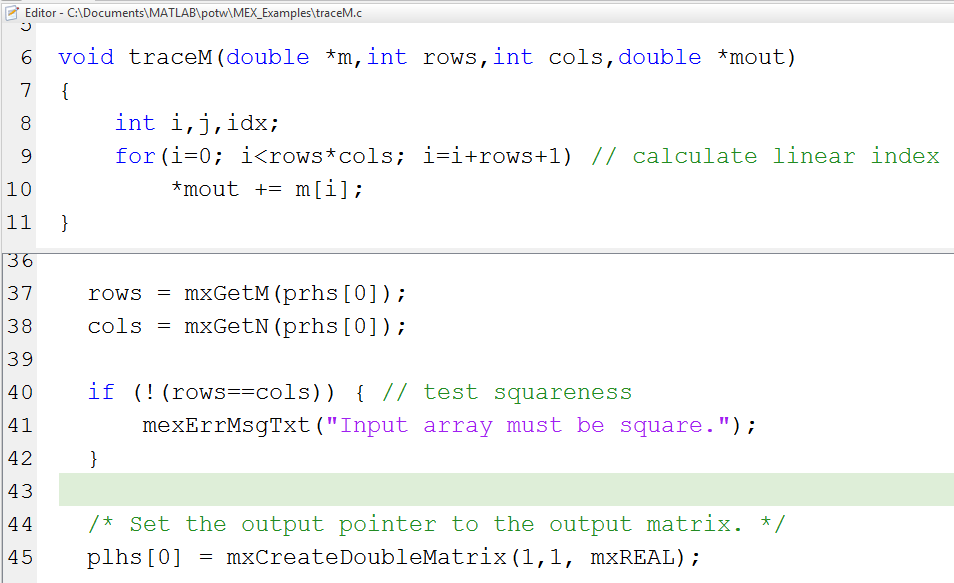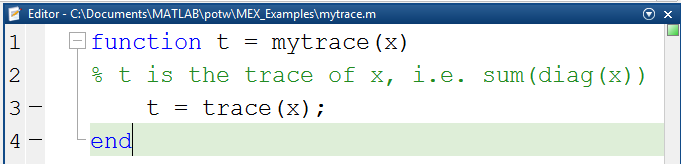# C-MEX Programming Tutorial Examples

Sean's pick this week is "C-MEX Programming Tutorial Examples" by Ilias Konsoulas.

I spent the last few days at the 2014 SIAM conference in Chicago manning the MathWorks booth with a few of our developers. We were asked many questions about the products, new features, life at MathWorks, and of course for t-shirts and rubik's cubes.

One of the questions I was asked was along the lines of: "How do I get started with MEX?". MEX, which is short for "MATLAB Executable", is compiled C/C++ or Fortran code with the appropriate MATLAB wrappers on it so that it can be called at the command line like any other MATLAB function. Personally, I haven't written any productive C/C++ code since high school about ten years ago. When I need C or C++ code, I develop the algorithm in MATLAB and then use MATLAB Coder to automagically generate equivalent C code.

I had found Ilias' submission a while ago and added it to the "to experiment with list". Now, it allowed me to answer this question while sounding knowledgable and getting the user started on his journey.

The submission comes with nine heavily commented C files that do simple matrix manipulations like summing and reshaping as well as a readme file that documents the package. The C files show the MEX syntax, required headers and some good programming practices like error checking.

Curious if I could actually write my own hand-written MEX file, I challenged myself to write one which calculates the sum of the diagonal of a matrix. I started by editing Ilias' transposeM.c which should be a good blueprint.

After some time, having it fail to compile 20ish times, and getting stuck in an infinite loop only once(!), (I forgot to increment i in the for-loop), it works!

Here's a snapshot of the file:That was an exercise in frustration. How about we just use MATLAB Coder to do this? First, I wrote a MATLAB function to calculate the sum of the diagonal; there happens to be a function called trace that does this:Now to generate C code, you can use the MATLAB Coder App to generate code interactively, or do it programatically with codegen. I'll take the latter approach since it's easier to automate.

% Input must be a double precision matrix of size "up-to-inf" by "up-to-inf"
input_specs = coder.typeof(zeros,inf,inf);
codegen mytrace -args {input_specs}

This generates mytrace_mex which I can call like any other function.

rng(5) % reproducible
X = gallery('randhess',7); % a matrix
t = mytrace_mex(X); % use mex file
disp(t)
   -0.1573


Now let's see if the two C implementations and the MATLAB implementation agree using the MATLAB unit testing framework.

% Build interactive test object
Tester = matlab.unittest.TestCase.forInteractiveUse;

% Assert equality
assertEqual(Tester,trace(X),traceM(X)) % My C implementation
assertEqual(Tester,trace(X),mytrace_mex(X)) % MATLAB Coder's implementation
Interactive assertion passed.
Interactive assertion passed.


### Comments

Give it a try and let us know what you think here or leave a comment for Ilias.

Published with MATLAB® R2014a

|

### Comments

To leave a comment, please click here to sign in to your MathWorks Account or create a new one.